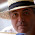## 10 August 2014

### Quiz 5: Print Fibonacci series till N numbers.

Problem:
Print Fibonacci series till N numbers.

Sample Input:
5

Sample Output:
First 5 elements of Fibonacci series are: 1 1 2 3 5

Solution:

my \$a = 1;
my \$b = 0;
my \$c = 0;
print "Enter the number of elements you want in fibonaaci series\n";
my \$num = <STDIN>;
chomp(\$num);
print "First \$num elements of fibonacci series : ";
for (my \$i=1; \$i <= \$num; \$i++)
{
\$c = \$a + \$b;
print "\$c ";
\$a = \$b;
\$b = \$c;
}

Tips:
• print "\$c ", here a space character is used to print all elements in 1 line and separated by a space.

#### 4 comments:

1.sub fibonacci {
my \$n = shift;

return undef if \$n < 0;
return 0 if \$n == 0;
return 1 if \$n == 1;
return fibonacci(\$n-1) + fibonacci(\$n-2);
}

print "Enter the number of elements you want in fibonaaci series: ";
chomp(my \$num = );
print "First \$num elements of fibonacci series : ";
print join ' ', map { fibonacci(\$_) } 0 .. \$num;
print "\n";

1.@Dave Cross: your solution gives result 0 1 1 2 3 5 for num=5

2.another method :

#!/usr/bin/perl -w
use strict;
print "Please enter N for fibonicci series:";
my \$input = ;
my @string = qw(1);
my \$fib = "0";
for(my \$i=0;\$i<\$input-1;\$i++)
{
\$fib = \$fib+\$string[\$i-1];
push(@string,\$fib);
}
chomp (@string);
print "@string\n";

1.@Santosh: Good, but for 0, your solution will give 1